#DAY 13
0
AI & Data

## 二、標準化方法

### 1. 正規化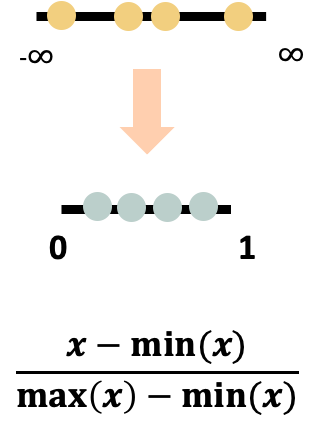``````train=pd.read_csv("train.csv")
y=pd.DataFrame(train["pm2.5"])
from sklearn.preprocessing import MinMaxScaler
scaler = MinMaxScaler()
y_train_scaled = scaler.fit_transform(y)
train_scaled = scaler_two.fit_transform(train)
train_scaled=pd.DataFrame(train_scaled,columns = ['month', 'day', 'hour', 'pm2.5', 'DEWP', 'TEMP', 'PRES', 'Iws', 'Is','Ir', 'cbwd_NE', 'cbwd_NW', 'cbwd_SE', 'cbwd_cv', 'year_2010',
'year_2011', 'year_2012', 'year_2013', 'year_2014'])
``````### 2. 標準化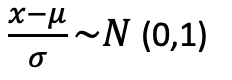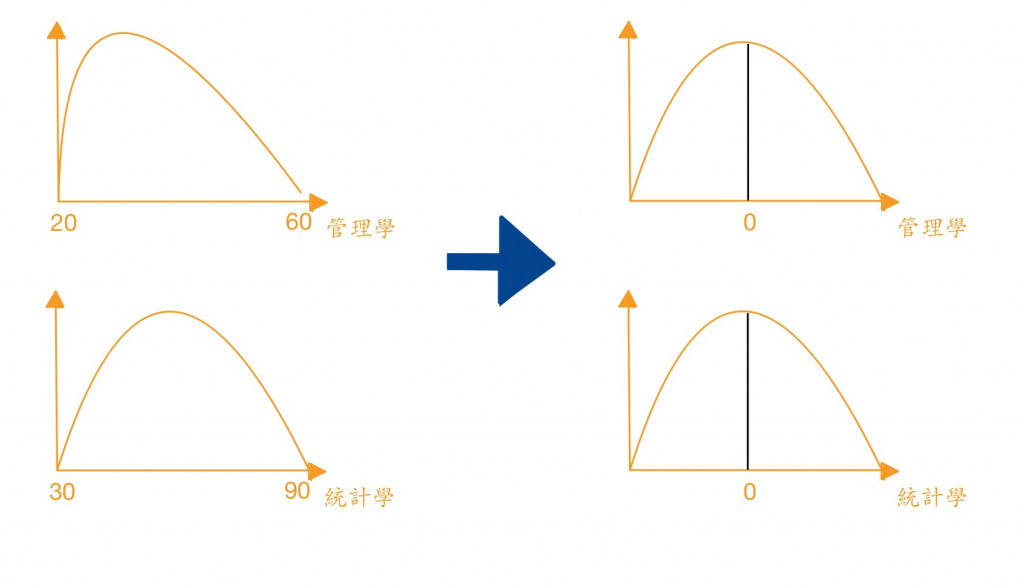``````def normalize(x_train, x_test, method = 'StandardScaler'):
'''
method = 'StandardScaler', 'PowerTransformer', 'MinMaxScaler'
'''
if method == 'StandardScaler':
normalize_function = preprocessing.StandardScaler()
if method == 'PowerTransformer':
normalize_function = preprocessing.PowerTransformer(method='yeo-johnson', standardize=True) # yeo-johnson, box-cox
if method == 'MinMaxScaler':
normalize_function = preprocessing.MinMaxScaler()

features = x_train.columns
for feature in features:
reshape = np.array(x_train[feature]).reshape(-1, 1)
x_train[feature] = normalize_function.fit_transform(reshape)

reshape = np.array(x_test[feature]).reshape(-1, 1)
x_test[feature] = normalize_function.transform(reshape)

return x_train, x_test

right_ratio_x_train, right_ratio_x_test = normalize(right_ratio_x_train, right_ratio_x_test)
``````

### 3. log轉換

``````log_df = np.log1p(df["你要轉換的資料名稱"])
``````

## 四、降維的方法

### 1. LDA

``````import numpy as np
import pandas as pd
from sklearn import datasets
#載入資料集
iris.keys()
X = pd.DataFrame(iris.data, columns=iris.feature_names)
y = iris.target
#使用LDA將資料變成二維
from sklearn.discriminant_analysis import LinearDiscriminantAnalysis
lda = LinearDiscriminantAnalysis(n_components=2)#將資料縮減成兩個維度
X_lda = lda.fit_transform(X, y)

lda.explained_variance_ratio_
``````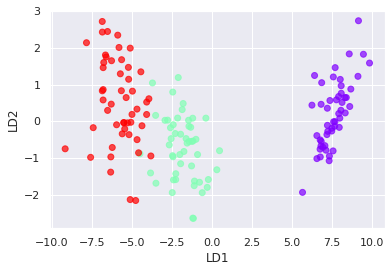### 底下我們用內建資料集資料鳶尾花實作

``````import numpy as np
import pandas as pd
from sklearn import datasets
#載入資料集
iris.keys()
X = pd.DataFrame(iris.data, columns=iris.feature_names)
y = iris.target
from sklearn.decomposition import PCA
pca = PCA(n_components=2)
X_pca = pca.fit_transform(X_train)
plt.xlabel('PC1')
plt.ylabel('PC2')
plt.scatter(
X_pca[:,0],
X_pca[:,1],
c=y_train,
cmap='rainbow',
alpha=0.7,
edgecolors='b')
``````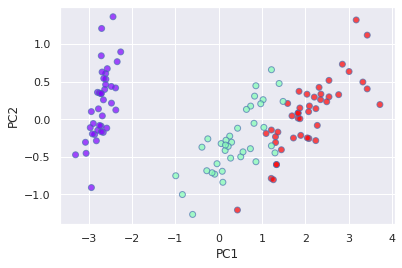### 3. LDA和PCA差別

PCA 是一種非監督式算法，其目標是找到資料集中的主成分之間最大的變異數。
LDA 是一種監督式的半別方法，將資料投影到軸上。可以形成特徵內彼此之間最小差異，與不同特徵最大差異。